# Above water surface

If we remove the stone from the water, we apply a force of 120N. How much force will we have to exert if we move the stone above the water surface? The density of the stone is 5000 kg/m ^ 3.

F2 =  150 N

### Step-by-step explanation: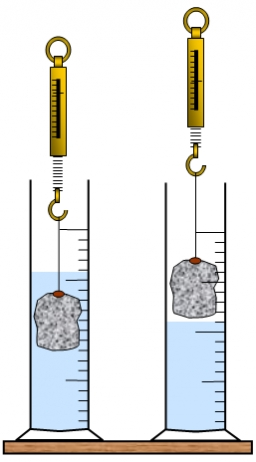Did you find an error or inaccuracy? Feel free to write us. Thank you!Tips to related online calculators
Do you know the volume and unit volume, and want to convert volume units?
Tip: Our Density units converter will help you with the conversion of density units.
Do you want to convert mass units?

## Related math problems and questions:

• A stone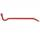There is a stone weighing 60 kg on the billet. The distance from the support point to the stone is 20cm. The length of the billet is 1m. Determine the force exerted by the hand at the end of the billet.
• Hollow sphereThe steel hollow sphere floats on the water plunged into half its volume. Determine the outer radius of the sphere and wall thickness, if you know that the weight of the sphere is 0.5 kg and the density of steel is 7850 kg/m3
• Hydrostatic forceWhat is the hydrostatic force applied to an area of 30 cm2 in the water at a depth of 20 m? (Water density is 1000 kg/m3)
• Dynamometer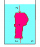What is the volume of a body that stretches a dynamometer in air, on which it is suspended by a force of 2.5 N, and if it is immersed in alcohol with a density of 800 kg/m ^ 3, does it tension the dynamometer with a force of 1.3 N?
• Heat transferWe placed a lead object weighing 0.4 kg and 250°C in 0.4 L water. What was the initial temperature of water t2 if the object's temperature and the water after reaching equilibrium was 35°C? We assume that the heat exchange occurred only between the lead o
• Floating of wood - Archimedes lawWhat will be the volume of the floating part of a wooden (balsa) block with a density of 200 kg/m3 and a volume of 0.02 m3 that floats in alcohol? (alcohol density is 789 kg/m3)
• The carThe car weight 1280 kg, increased its speed from 7.3 m/s to 63 km/h on a track of 37.2 m. What force did the car engine have to exert?
• Reservoir + waterThe reservoir filled with water weighs 12 kg and after pouring off three-quarters of the water weights 3 kg. Calculate the weight and volume of the reservoir.
• Cuboid 5Calculate the mass of the cuboid with dimensions of 12 cm; 0.8 dm and 100 mm made from spruce wood (density = 550 kg/m3).
• A steel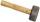A steel hammer with a weight of 0.5 kg hits the nail at a speed of 3 m/s. How deep the nail penetrates the wood if the hammer acts on the nail with an average force of 50N.
• Iron sphereIron sphere has weight 100 kg and density ρ = 7600 kg/m3. Calculate the volume, surface, and diameter of the sphere.
• Aluminum wireAluminum wire of 3 mm diameter has a total weight of 1909 kg and a density of 2700 kg/m3. How long is the wire bundle?What overload in g (g-force) has passed the pilot if he accelerated from 0 to 600 km/h in 3 seconds?If a person weighs 80 kg, how many kilograms of cork must take a swimming belt to float on water? The density of the human body is 1050kg/m3 and cork 300kg/m3. (Instructions: Let the human body and cork on a mixture with a density of 1000kg/m3. When you m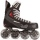Two boys weighing 60kg and 90kg move on roller skates and are attracted to each other by a rope. One boy exerts a force of 15N. What force does the other boy exert? What accelerations will the boys gain? Do not consider friction or air resistance.Mixing 62 kg of sea water with 84 kg rainwater is created water containing 3.1% salt. How many percent sea water contains salt?The maximum weight of the car is 5000 kg. 10 m3 of sand must be transferred. How many times does a car have to go? (density of sand is 1500 kg/m3)